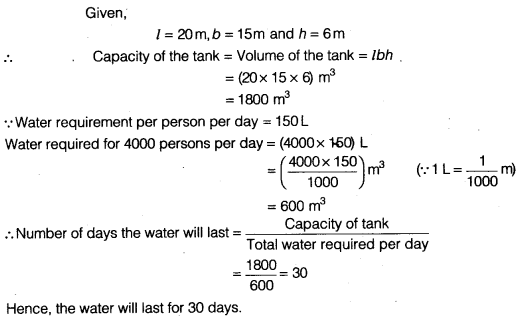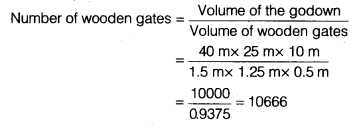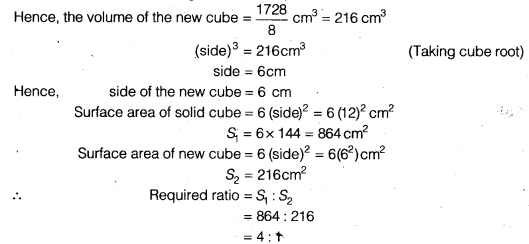NCERT Solutions for Class 9 Maths Chapter 13 Surface Areas and Volumes Ex 13.5 are part of NCERT Solutions for Class 9 Maths. Here we have given NCERT Solutions for Class 9 Maths Chapter 13 Surface Areas and Volumes Ex 13.5.

 Board CBSE Textbook NCERT Class Class 9 Subject Maths Chapter Chapter 13 Chapter Name Surface Areas and Volumes Exercise Ex 13.5 Number of Questions Solved 9 Category NCERT Solutions

## NCERT Solutions for Class 9 Maths Chapter 13 Surface Areas and Volumes Ex 13.5

Question 1.
A matchbox measures 4 cm x 2.5 cm x 1.5 cm. What will be the volume of a packet containing 12 such boxes?
Solution:
Volume of a match box = 4 cm x 2.5 cm x 1.5 cm= 15 cm3
Volume of a packet = 12 x 15 cm3 = 180 cm3

Question 2.
A cuboidal water tank is 6 m long, 5 m wide and 4.5 m deep. How many litres of water can it hold? ( 1 m3 = 1000L)
Solution:
Volume of a cuboidal water tank = 6 m x 5 m x 4.5 m
= 30 x 4.5 m3 = 135 m3
= 135 x 1000L = 135000L (∵ 1 m3 = 1000L)

Question 3.
A cuboidal vessel is 10 m long and 8 m wide. How high must it be made to hold 380 cubic metres of a liquid?
Solution:
Volume of a cuboidal vessel = hold liquid
∴ l x b x h = 380 m3
⇒ 10 x 8 x h = 380
⇒ h = $$\frac { 380 }{ 80 }$$
⇒ h= 4.75m
Hence, the cuboidal vessel must be made 4.75 m high.

Question 4.
Find the cost of digging a cuboidal pit 8 m long, 6 m broad and 3 m deep at the rate of ₹30 per m3.
Solution:
Volume of a cuboidal pit = l x bx h= (8 x 6 x 3)m3 =144 m3
∵ Cost of digging per m3 = ₹ 30
∴ Cost of digging 144m3 = ₹ 30x 144 = ₹ 4320

Question 5.
The capacity of a cuboidal tank is 50000 litres of water. Find the breadth of the tank, if its length and depth are 2.5 m and 10 m, respectively.
Solution:
Given, l = 2.5 m and h = 10m
Let breadth of tank be b.Hence, breadth of the cuboidal tank is 2 m.

Question 6.
A village, having a population of 4000, requires 150 litres of water per head per day. It has a tank measuring 20 m x 15 m x 6 m. For how many days will the water of this tank last?
Solution:Question 7.
A go down measures 40 m x 25 m x 10 m. Find the maximum number of wooden crates each measuring 15 m x 125 m x 0.5 m that can be stored in the go down.
Solution:
Dimension for godown, l = 40m b = 25 m and c = 10 m
Volume of the godown = l x b x h= 40 m x 25 m x 10 m
Dimension for wooden gate l = 1.5 m, b = 1.25 m, b = 1.25 m, h = 0.5 m
Volume of wooden gates = l x b x h = 1.5 m x 1.25 m x 0.5mQuestion 8.
A solid cube of side 12 cm is cut into eight cubes of equal volume. What will be the side of the new cube? Also, find the ratio between their surface areas.
Solution:
Volume of a solid cube = 12 cm x 12 cm x 12 cm = 1728 cm3
The solid cube is cut into eight cubes of equal volume.Question 9.
A river 3 m deep and 40 m wide is flowing at the rate of 2 km per hour. How much water will fall into the sea in a minute?
Solution:
Given, l = 2 km= 2 x 1000 m = 2000m
b = 40 m
and h = 3 m
Since, the water flows at the rate of 2 km h-1,
i.e., the water from 2 km of river flows into the sea in one hour.
The volume of water flowing into the sea in one hour = Volume of the cuboid
= l x b x h
= (2000 x 40 x 3) m3
= 240000 m3
∴ The volume of water flowing into the sea in one minute
= $$\frac { 240000 }{ 60 }$$ m3
= 4000 m3

We hope the NCERT Solutions for Class 9 Maths Chapter 13 Surface Areas and Volumes Ex 13.5 help you. If you have any query regarding NCERT Solutions for Class 9 Maths Chapter 13 Surface Areas and Volumes Ex 13.5, drop a comment below and we will get back to you at the earliest.